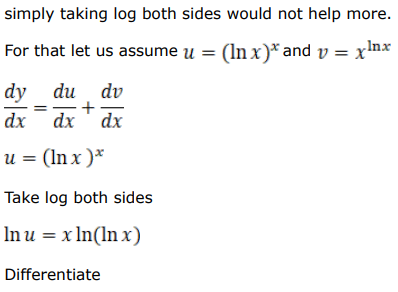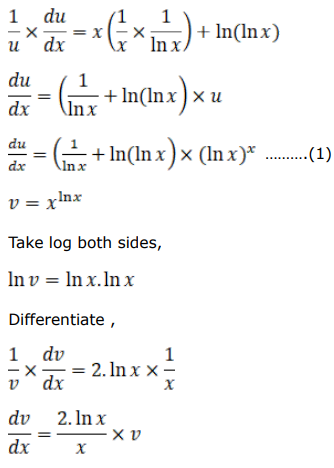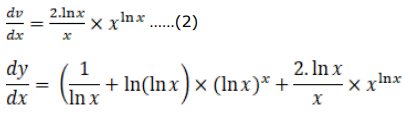# Solve this followingQuestion:

Find $\frac{d y}{d x}$, when:

$y=(\log x)^{x}+x^{\log x}$

Solution: